# Algebraic Expressions Questions and Answers - Form 1 Topical Mathematics

## Questions

1. Five years ago, a mother’s age was four times that of her daughter. In four years to come, she will be 2 ½ times the age of her daughter. Calculate the sum of their present ages
2. Simplify;
1. 6a – 2b + 7a – 4b + 2

2.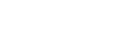3. Simplify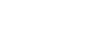4. Given that x + y = 8 and x2 + y2 = 34
Find;
1. the value of x2 + 2xy + y2
2. Find the value of ; 2xy
3. x2 – 2xy + y2
4. Value of x and y
5. Simplify the expression.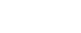6. Simplify the expression
2/3(3x -2) – 3/4(2x -2)
7. Simplify by factorizing completely: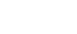8. Simplify as far as possible.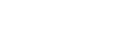9. Simplify:

1.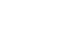2. hence solve:-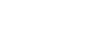10. Factorize completely the expression
75x2 – 27y2
11. Simplify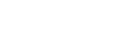12. Simplify the expression:-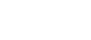13. Given that (x-3) (Ax2+bx+c) = x3-7x-6, find the value of A, B and C
14.
1. solve for y in 8x(22)y=6x2y-1
2. Simplify completely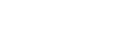15. Simplify the expression.: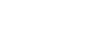16. Simplify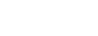17. The sum of two numbers is 15. The difference between five times the first number and three times the second number is 19. Find the two numbers.
18. Simplify the following expressions by reducing it to a single fraction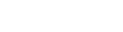19. Simplify the expression:-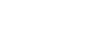1. Let the daughter’s age 5yrs ago be x
Mother 4x
come;
Daughter = x + 9
Mother = 4x+ 9
4x + 9 = 5/2(x +9)
4x + 9 = 2.5x + 22.5
1.5x = 13.5
x = 9
Mother = 41yrs
14 + 41 =55
2.
1. 6a + 7a – 2b – 4b + 2
= 13a – 6b + 2

2.3.4.
1. From x + y and x2 + y2 = 34
X = 8 – y
Substituting for x in x2 – y2 = 34
(8 – y) (8 – y) + y2 = 34
64 – 8y – 8y + y2 + y2 = 34
64 – 16y + 2y2 = 34
2y2 – 16y + 64 – 34 = 0
2y2 – 16y + 30 = 0
y2 = 8y + 15 = 0
y (y – 3) – 5 (y-3) = 0 (y-5) (y – 3)
y is either 5 or 3
but x – y = 8
x is either 5 0r 3
x2 + 2xy + y2 = 32 + 2 x 3 x 5 + 25
= 9 + 30 + 25 = 64
2. 2xy = 2 x 3 x 5 = 30
3. x2 – 2xy + y2 = 9 – 2 x 3 x 5 + 25 = 4
4. x - y = 8 and x2 + y2 = 34
x = 8 – y
(8 – y)2 + y2 = 34
y2 – 8y + 15 = 0
y2 – 3y – 5y + 15 = 0
y(y -3) – 5(y – 3)
(y-3) = 0 y = 3
(y-5) = 0 y = 5
x + 3 = 8, x = 5 or x + 5 = 8
x = 3
x is either 3 or 5
y is either 3 or 5

5.6.7.8.9.10.  3( 25x2 – 9y2)
3(5x + 3y)(5x – 3y)
11. Factorizing the numerator
= p(p2 – q2) + q(p2-q)
= (p+q) (p2-q2)
= (p+q) (p+q) n(p-q)
Factorising the denominator
(p+q) (p+q)
Numerator = p - q
Denominator
12. ( 3x + 2y ) ( 3x - 2y )
( 3x + 2y ) ( 3x - 2y )
3x + 2y
4x + 3y
13. (x – 3) (AX2 +BX + C) = x3 – 7x – 6
AX3 + BX2 +CX – 3AX2 – 3BX – 3c = x3 – 7x – 6
A = 1
B – 3A = 0
B – 3 x 1 = 0
B = 3
-3c = -6
c = 2
14.
1. 8(22)y = 6 x 2y – 1
let t = 2y
8t2 = 6t – 1
8t2 – 4t – 2t + 1 = 0
(4t – 1) (2t – 1) = 0
t = ¼ or ½
t = 2y = ¼ = 2-2
y = -2
or t = 2y = ½ = 2-1
y = -1
y = -2 or -1

2.15.16.17. Let the numbers be a and b
a + b = 15 x3
5a – 3b = 19 x 1

3a + 3b = 45
5a – 3b = 19
8a = 64
a = 8
b = 7

18.19.• ✔ To read offline at any time.
• ✔ To Print at your convenience
• ✔ Share Easily with Friends / Students